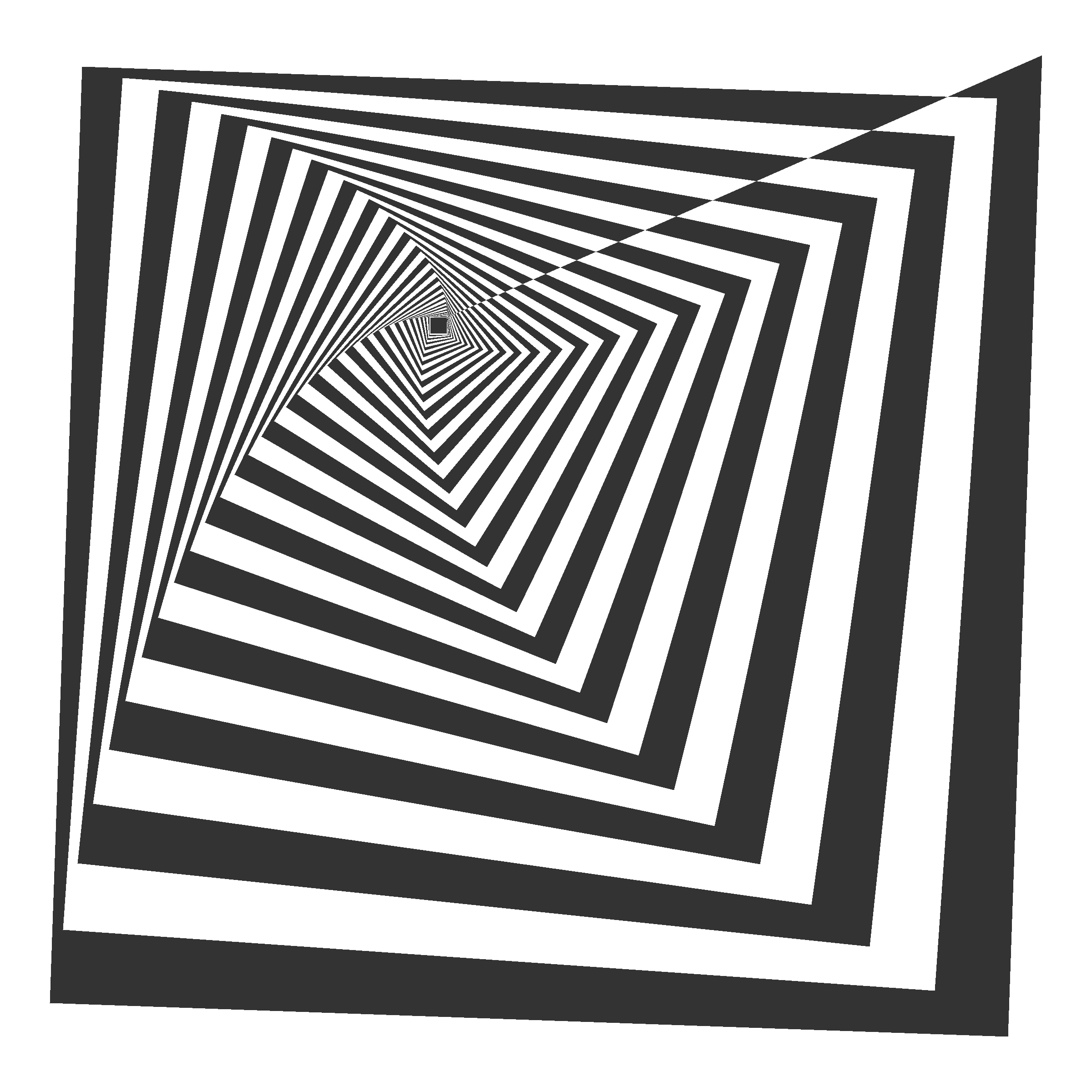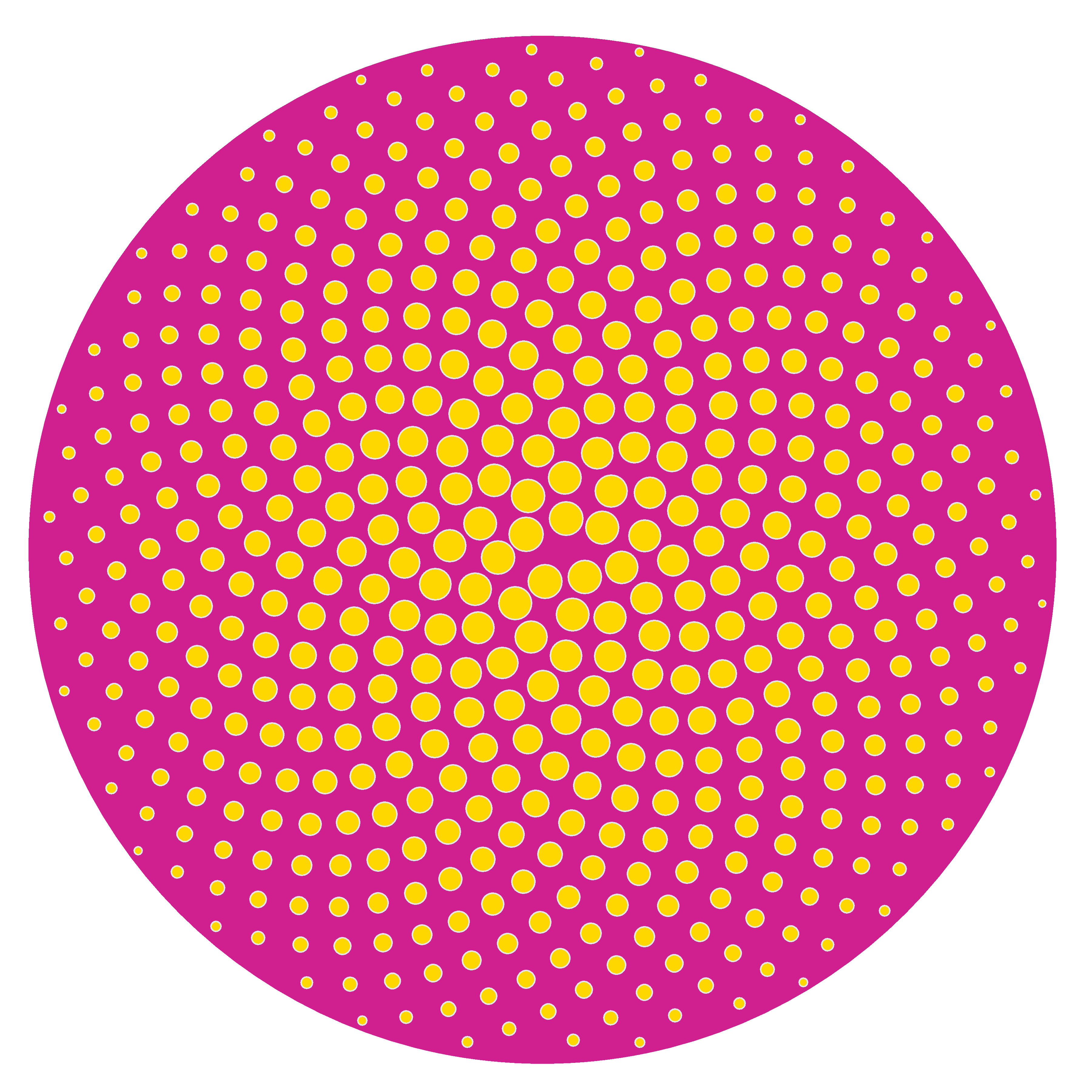# Tweetable Mathematical Art With R

Sin ese peso ya no hay gravedad
Sin gravedad ya no hay anzuelo
(Mira cómo vuelo, Miss Caffeina)

I love messing around with R to generate mathematical patterns. I always get surprised doing it and gives me lot of satisfaction. I also learn lot of things doing it: not only about R, but also about mathematics. It is one of my favourite hobbies. Some time ago, I published this post showing some drawings, each of them generated with less than 280 characters of code, to be shared on Twitter. This post came to appear in Hacker News, which provoked an incredible peak on visits to my blog. Some comments in the Hacker News entry are very interesting.

This Summer I delved into this concept of Tweetable Art publishing several drawings together with the R code to generate them. In this post I will show some.

Vertiginous Spiral

I came up with this image inspired by this nice pattern. It is a turtle graphic inspired pattern but instead of drawing lines I use geom_polygon to colour the resulting image in black and white:Code:

```library(tidyverse)
df <- data.frame(x=0, y=0)
for (i in 2:500){
df[i,1] <- df[i-1,1]+((0.98)^i)*cos(i)
df[i,2] <- df[i-1,2]+((0.98)^i)*sin(i)
}
ggplot(df, aes(x,y)) +
geom_polygon()+
theme_void()
```

Slight modifications of the code can generate appealing patterns like this:Marine Creature

A combination of sines and cosines. It reminds me a jellyfish:Code:

```library(tidyverse)
seq(from=-10, to=10, by = 0.05) %>%
expand.grid(x=., y=.) %>%
ggplot(aes(x=(x^2+pi*cos(y)^2), y=(y+pi*sin(x)))) +
geom_point(alpha=.1, shape=20, size=1, color="black")+
theme_void()+coord_fixed()
```

Summoning Cthulhu

The name is inspired in an answer from Mara Averick to this tweet. It is a modification of the marine creature in polar coordinates:Code:

```library(tidyverse)
seq(-3,3,by=.01) %>%
expand.grid(x=., y=.) %>%
ggplot(aes(x=(x^3-sin(y^2)), y=(y^3-cos(x^2)))) +
geom_point(alpha=.1, shape=20, size=0, color="white")+
theme_void()+
coord_fixed()+
theme(panel.background = element_rect(fill="black"))+
coord_polar()
```

Naive Sunflower

Sunflowers arrange their seeds according a mathematical pattern called phyllotaxis, whic inspires this image. If you want to create your own flowers, you can do this Datacamp’s project. It’s free and will introduce you to the amazing world of `ggplot2`, my favourite package to create images:Code:

```library(ggplot2)
a=pi*(3-sqrt(5))
n=500
ggplot(data.frame(r=sqrt(1:n),t=(1:n)*a),
aes(x=r*cos(t),y=r*sin(t)))+
geom_point(aes(x=0,y=0),
size=190,
colour="violetred")+
geom_point(aes(size=(n-r)),
shape=21,fill="gold",
colour="gray90")+
theme_void()+theme(legend.position="none")
```

Silk Knitting

It is inspired by this other pattern. A lot of almost transparent white points ondulating according to sines and cosines on a dark coloured background:Code:

```library(tidyverse)
seq(-10, 10, by = .05) %>%
expand.grid(x=., y=.) %>%
ggplot(aes(x=(x+sin(y)), y=(y+cos(x)))) +
geom_point(alpha=.1, shape=20, size=0, color="white")+
theme_void()+
coord_fixed()+
theme(panel.background = element_rect(fill="violetred4"))
```

Try to modify them and generate your own patterns: it is a very funny way to learn R.

Note: in order to make them better readable, some of the pieces of code below may have more than 280 characters but removing unnecessary characters (blanks or carriage return) you can reduce them to make them tweetable.

## 8 thoughts on “Tweetable Mathematical Art With R”

1.Forest Wussten says:

Very interesting post. However, I ran into some problem replicating the first example.. The code posted will give me a solid polygon without the white “holes”. I am not sure how the geom_polygon was filled with different colors..

2.Chen says:

The codes for the first graph do not work.

1.@aschinchon says:

Try to change slighly the 0.98 parameter

2.Forest Wussten says:

I think it depends on the version of ggplot2. The first plot can be plotted without issue in older version but not in 3.0.0+.

3.Fan says:

try use an older version of ggplot2.

3.Shornone says:

These are great. I am new to R and find the potential exciting but a bit overwhelming also.

Why use points instead of paths? If the Marine Creature was made of lines it would be more or less ready to use with a plotter.

1.@aschinchon says:

Thank you. I think it’s not as simple as it seems since to use lines (geom_path), the points must be arranged “ready to be connected” and this property is not guaranteed by the way I use to generate them. I will try it anyway.

1.Shorn says:

I was able to make it work. I sent you the tweet